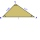# Harmonic mean + area - math problems

#### Number of problems found: 4

• Statistical surveyWrite TRUE OR FALSE for each question: 1 Standard deviation is a measure of central location. 2. The most frequent observation in a data set is known as the mode. 3 The most passive method of data collection is observation. 4 Access time for secondary dat
• Statistics quizFill in the missing word 1. in a data set, the mean, median and mode are measured of ________________ 2. "The manipulation of variables under controlled conditions"is the data collection method known as______________ 3. in a normal distribution, the area
• Three altitudesA triangle with altitudes 4; 5, and 6 cm is given. Calculate the lengths of all medians and all sides in a triangle.
• Right isosceles triangleRight isosceles triangle has an altitude x drawn from the right angle to the hypotenuse dividing it into 2 equal segments. The length of one segment is 5 cm. What is the area of the triangle?

We apologize, but in this category are not a lot of examples.
Do you have an exciting math question or word problem that you can't solve? Ask a question or post a math problem, and we can try to solve it.

We will send a solution to your e-mail address. Solved examples are also published here. Please enter the e-mail correctly and check whether you don't have a full mailbox.

Looking for calculator of harmonic mean? Harmonic mean - math problems. Area - math problems.